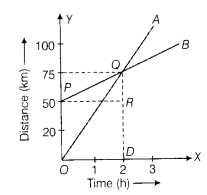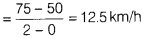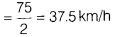# Distance-time graph of two trains

The distance-time graph of two trains are shown in figure. The trains start simultaneously in the same direction.1. How much is B ahead of A when the motion starts?
2. What is the speed of B?
3. When and where will A catch B?
4. What is the difference between speeds of A and B?
5. Is the speed of both the trains uniform or non-uniform? Justify your answer.
1. According to the situation in given graph, B is ahead of A by 50 km.
2. Speed of B = slope of PQ3. A and 6 meet at Q, i.e. 75 km from origin and 2h after the start.
4. Speed of A = slope of OQDifference between speeds = 37.5 - 12.5 = 25 km/h
5. Speed of both the trains is uniform as s-t graph makes a straight line.